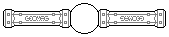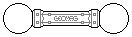Web textodigital.com
Around the rhombicosidodecahedron, 1
In Construction of the reinforced rhombicosidodecahedron, method 2, step 4, the stabilizing pentagons in each set of three mutually perpedicular pieces met in eight trihedral angles: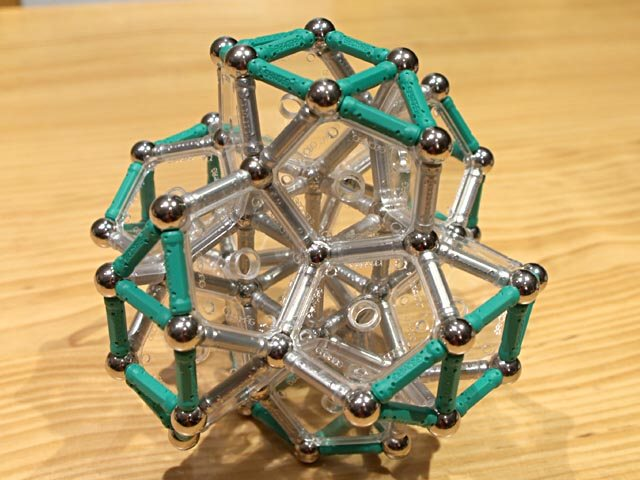Eight trihedral angles
These eight angles are identical to those at vertices in the regular dodecahedron. So, we could construct eight corresponding dodecahedra on them: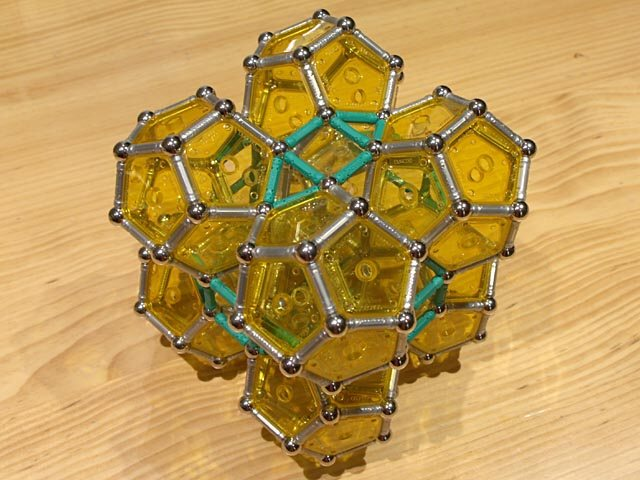Eight regular dodecahedra around a rhombicosidodecahedron 496 pieces: 136 balls, 264 rods, 96 pentagons (2.70 kg)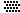This idea is further explored in which follows, but beginning with a finished reinforced rhombicosidodecahedron, as the previous structure is very weak. First, we complete all 12 regular icosahedra which can be built using the pyramids which stabilize the pentagonal faces of the object: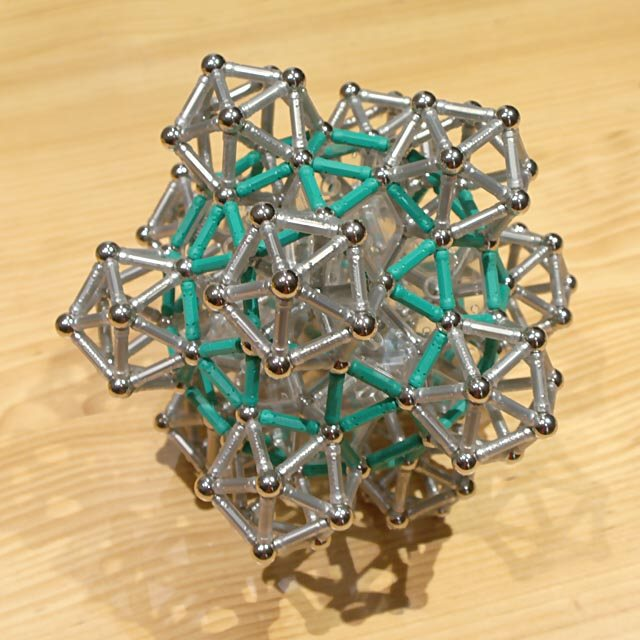Twelve regular icosahedra around a rhombicosidodecahedron 632 pieces: 152 balls, 456 rods, 24 pentagons (3.52 kg)If we build now the eight regular dodecahedra mentioned above, they will turn out intermingled with the icosahedra: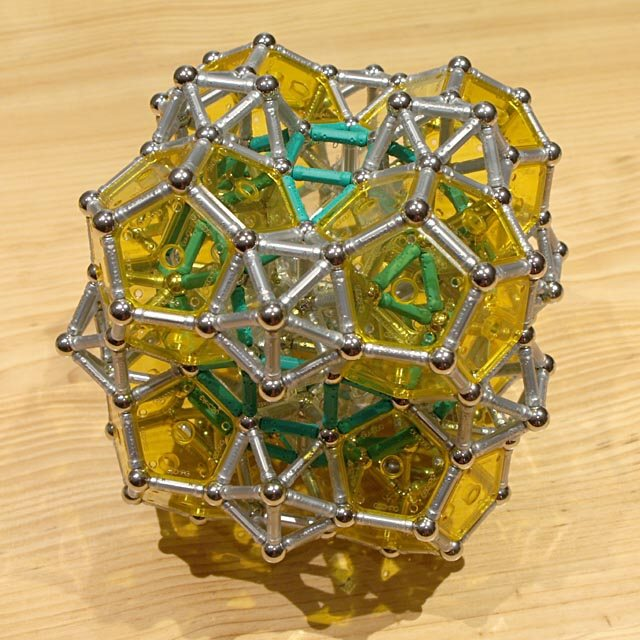Twelve icosahedra, and eight dodecahedra around a rhombicosidodecahedron 784 pieces: 184 balls, 528 rods, 72 pentagons (4.28 kg)As can be seen in the previous picture, each group of four dodecahedra and four icosahedra makes up a cradle, with the shape of two intersecting icosidodecahedra. In fact, six of these pairs could be added (although just three pairs were added for the following picture, the quantities of pieces given are the total required):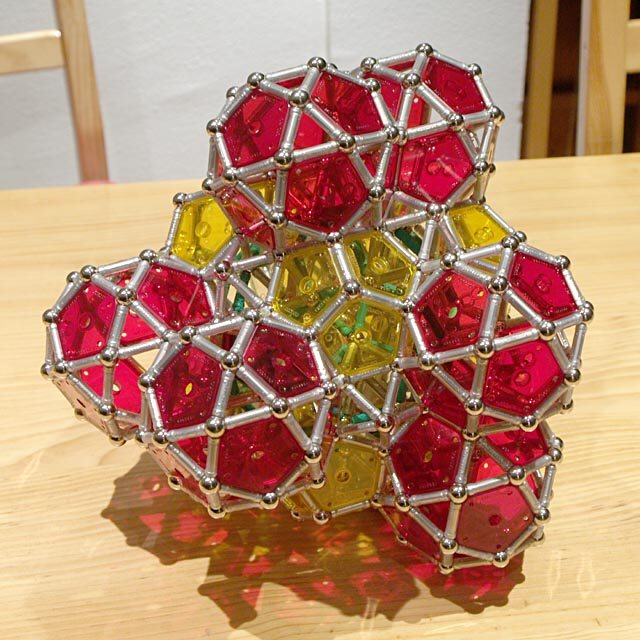Twelve icosahedra, eight dodecahedra, and twelve icosidodecahedra around a rhombicosidodecahedron (incomplete) 1612 pieces: 400 balls, 1032 rods, 180 pentagons (8.83 kg)The icosahedra which we added in step 1 are nearly buried under the bodies that we added afterwards. Only the pentagonal pyramids which are farthest from the center of the object remain visible, and they are surrounded by three pentagons (from three corresponding icosidodecahedra), and two holes with the right shape for fitting two more pentagons, all at the appropriate angles to build twelve new dodecahedra (again, we only built three of them for this picture, but the quantities given are the total required):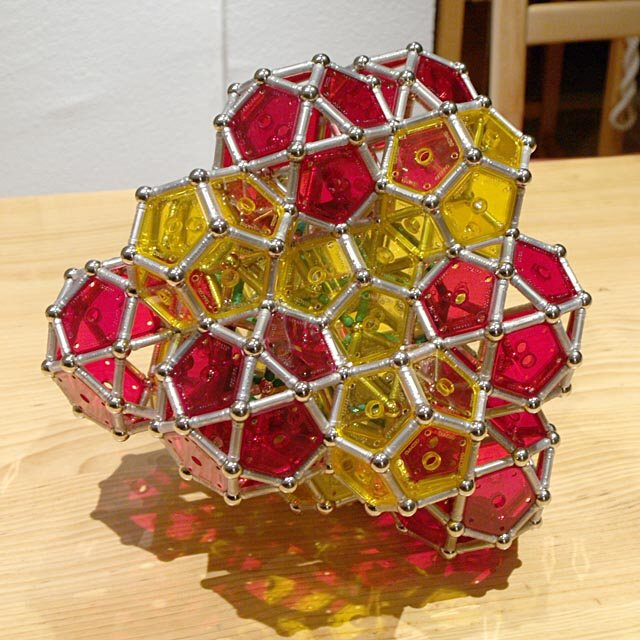Twelve icosahedra, eight dodecahedra, twelve icosidodecahedra, and another twelve dodecahedra around a rhombicosidodecahedron (incomplete) 1960 pieces: 484 balls, 1200 rods, 276 pentagons (10.63 kg)In each successive extension the object becomes heavier and weaker, and less easy to handle. In this case, we didn't even try completing the last two steps. But we cannot state that it cannot be done.